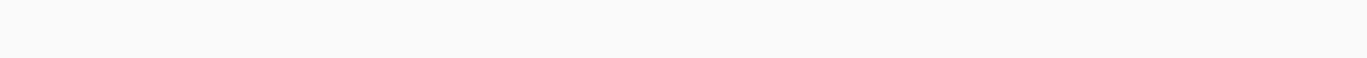# Vectorized Operations in NumPy with examples

In this tutorial, we will see the difference in the time taken between normal iterative operations and vectorized operations in NumPy in Python with examples. If we talk about normal iterative operations in Python, it normally takes a long time to give an output. Whereas, performing vectorized operations in NumPy in Python programming takes much lesser time than the normal iterative operation. So, let’s see the examples given below to understand and learn.

## Example 1:

```# import the necessary modules
import numpy as np
import timeit

# vectorize the sum using np.sum()
print(np.sum(np.arange(15000)))
#print the time taken by vectorized sum
print("Time taken by vectorized sum : ", end = "")
%timeit np.sum(np.arange(15000))

#  calculate the iterative sum using loop
total = 0
for i in range(0, 15000):
total += i
a = total
print("\n" + str(a))
#print the time taken by iterative sum
print("Time taken by iterative sum : ", end = "")
%timeit a
```

Output:Through this example, we see the output which shows the time taken between iterative and vectorized operations. In this program, the first step is to import the necessary libraries or modules, then vectorize the sum by using `np.sum()` and determine the range or interval to which the time should be calculated by using the NumPy `arange()` function in Python(which calculates sequences of numerical intervals) and print out the vectorized sum. This will then be followed by calculating the iterative sum using loops and the total value, and finally printing the iterative sum.

## Example 2:

```# import the following modules
import numpy as np
import timeit
import math

# calculate exponential operation and print the time taken by exponential operation
print("Time taken by exponential operation : ", end = "")
#calculte the time taken using np.arrange() function
%timeit np.exp(np.arange(170))

# calculate the non-exponential operation and print the time taken by non-exponential operation
print("Time taken by non-expotential operation : ", end = "")
#calculate the time taken using for loop in the specific range
%timeit [math.exp(i) for i in range(170)]```

Output:Another NumPy example that shows vectorized operations are faster than non-vectorized operations. The example illustrates the comparison between exponential and non-exponential functions in Python, which evaluates the exponential value of an object. Here we import the required libraries as stated above, then we calculate the time by exponential operation using the `np.arange()` function, and then we print the time. The next step is to calculate the time based on a non-exponential or simple operation. Loops are used for this calculation.